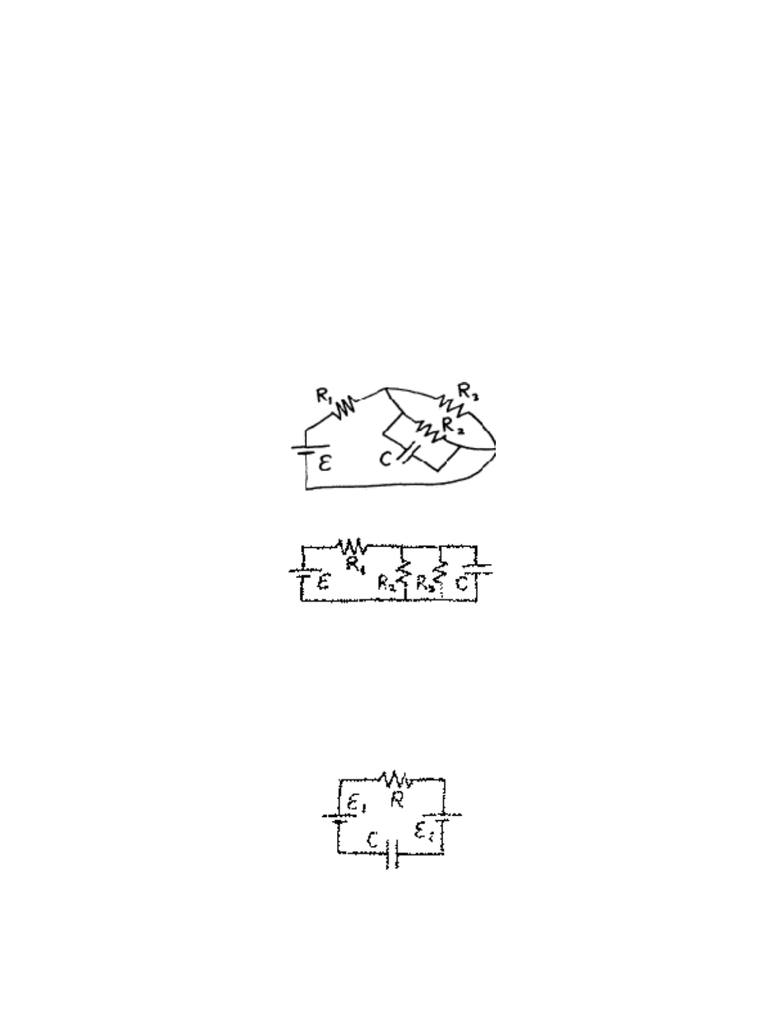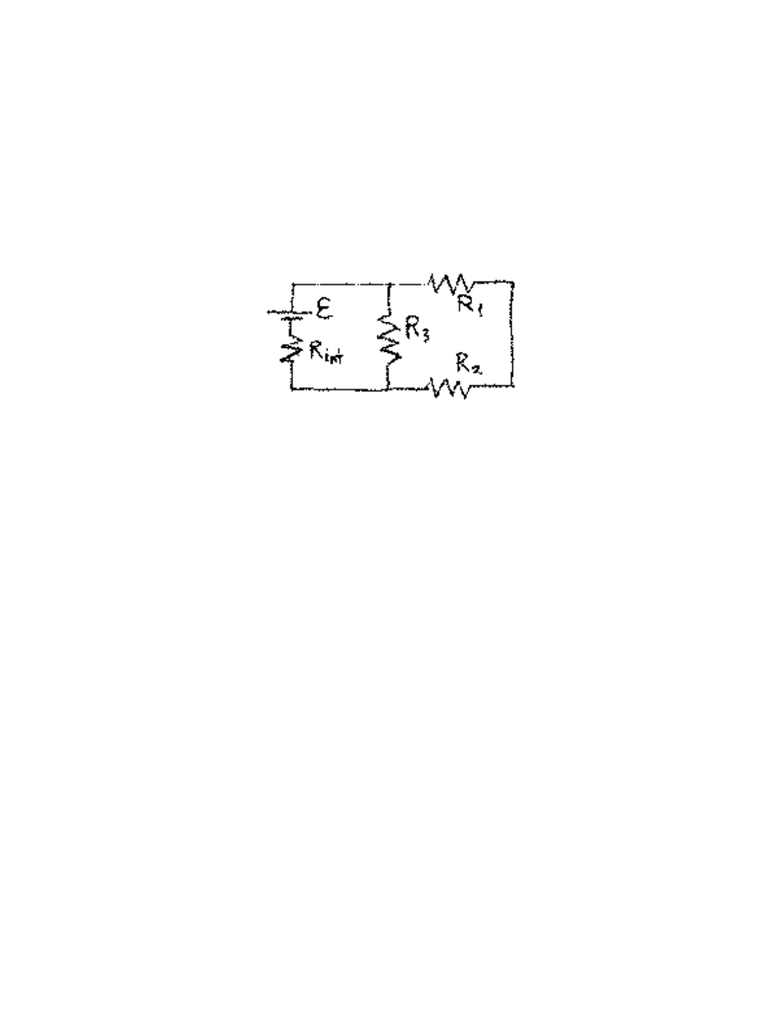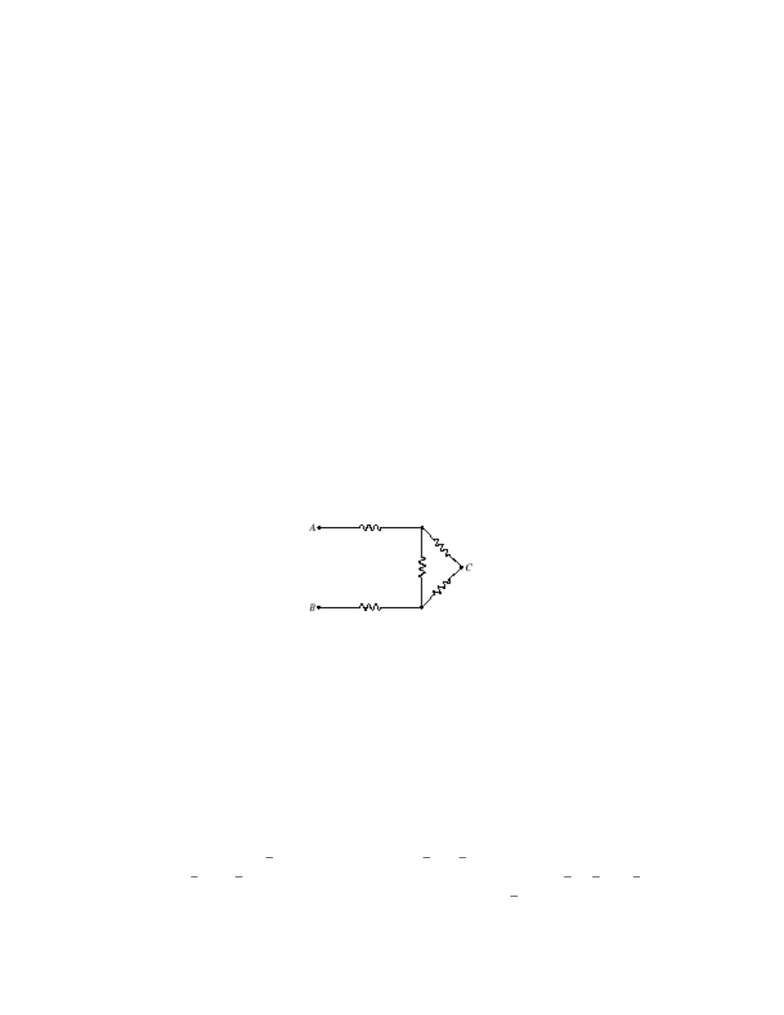# notes for physics

163 views24 pagesCHAPTER 28 ELECTRIC CIRCUITS
ActivPhysics can help with these problems: Section 12, “DC Circuits”
Section 28-1: Circuits and Symbols
Problem
1. Sketch a circuit diagram for a circuit that includes a resistor
R
1
connected to the positive terminal of a battery, a pair of
parallel resistors
R
R
2
3
and
connected to the lower-voltage end of
R
1
, then returned to the battery’s negative terminal,
and a capacitor across
R
2
.
Solution
A literal reading of the circuit specifications results in connections like those in sketch (a). Because the connecting wires are
assumed to have no resistance (a real wire is represented by a separate resistor), a topologically equivalent circuit diagram is
shown in sketch (b).
Problem 1 Solution (a).
Problem 1 Solution (b).
Problem
2. A circuit consists of two batteries, a resistor, and a capacitor, all in series. Sketch this circuit. Does the description
allow any flexibility in how you draw the circuit?
Solution
In a series circuit, the same current must flow through all elements. One possibility is shown. The order of elements and the
polarity of the battery connections are not specified.
Problem 2 Solution.
www.notesolution.com
Unlock document

This preview shows pages 1-3 of the document.
Unlock all 24 pages and 3 million more documents.660 CHAPTER 28
Problem
3. Resistors
R
1
and
R
2
are connected in series, and this series combination is in parallel with
R
3
. This parallel
combination is connected across a battery whose internal resistance is
R
int
.
Draw a diagram representing this circuit.
Solution
The circuit has three parallel branches: one with
R
1
and
R
2
in series; one with just
R
3
; and one with the battery (an ideal
emf in series with the internal resistance).
Problem 3 Solution.
Section 28-2: Electromotive Force
Problem
4. What is the emf of a battery that delivers 27 J of energy as it moves 3.0 C between its terminals?
Solution
From the definition of emf (as work per unit charge), E
=
=
W
q
= =
27
3
9
J
C
V
.
Problem
5. A 1.5-V battery stores 4.5 kJ of energy. How long can it light a flashlight bulb that draws 0.60 A?
Solution
The average power, supplied by the battery to the bulb, multiplied by the time equals the energy capacity of the battery. For
an ideal battery,
P
E
=
I
,
therefore
E
It
=
4
5
.
,
kJ
or t= = × =4 5 15 0 60 5 10 139
3
.(.)( .). kJ V A s h.=
Problem
6. If you accidentally leave your car headlights (current drain 5 A) on for an hour, how much of the 12-V battery’s
chemical energy is used up?
Solution
The power delivered by an emf is
E
I
,
so (if the voltage and current remain constant) the energy converted is
E
It
=
=
(
)(
)(
)
.
12
5
3600
216
V
A
s
kJ
Problem
7. A battery stores
50
W
h
of chemical energy. If it uses up this energy moving
3 0
10
4
.
×
C
through a circuit, what is its
voltage?
www.notesolution.com
Unlock document

This preview shows pages 1-3 of the document.
Unlock all 24 pages and 3 million more documents.CHAPTER 28 661
Solution
The emf is the energy (work done going through the source from the negative to the positive terminal) per unit charge:
E=× =()( ) ( ) .50 3600 3 10 6
4
W h s/h C V= (This is the average emf; the actual emf may vary with time.)
Section 28-3: Simple Circuits: Series and Parallel Resistors
Problem
8. A
47-
k
resistor and a
39-
k
resistor are in parallel, and the pair is in series with a
22-
k
resistor. What is the
resistance of the combination?
Solution
From Equations 28-1 and 3c,
R
=
+
+
=
22
47
39
47
39
43
3
k
k
k
(
)
(
)
.
.
=
Problem
9. What resistance should be placed in parallel with a
56-
k
resistor to make an equivalent resistance of
45 k
?
Solution
The solution for R2 in Equation 28-3a is
R
R
R
R
R
2 1 1 56 45 56 45 229
=
=
=
parallel parallel k k .= =( ) ( )( ) ( )
Problem
10. In Fig. 28-49 all resistors have the same value, R. What will be the resistance measured (a) between A and B or
(b) between A and C?
FIGURE 28-49 Problems 10 and 11.
Solution
(a) The resistance between A and B is equivalent to two resistors of value R in series with the parallel combination of
resistors of values R and 2R. Thus,
R
R
R
R
R
R
R
R
AB
=
+
+
+
=
(
)
(
)
.
2
2
8
3
= = (b)
R
AC
is equivalent to just one resistor of
value R in series with the parallel combination of R and 2R (since the resistor at point B carries no current, i.e., its branch is
an open circuit). Thus
R
R
R
R
R
R
AC
=
+
=
(
)
.
2
3
5
3
= =
Problem
11. In Fig. 28-49, take all resistors to be
10.
. If a 6.0-V battery is connected between points A and B, what will be the
current in the vertical resistor?
Solution
The circuit in Fig. 28-49, with a battery connected across points A and B, is similar to the circuit analysed in Example 28-4.
In this case, R|| ()( ) ( ) ( ) ,= + =1 2 1 2 2
3
Ω Ω= and Rtot = + + =1 1 2
38
3
Ω Ω . The total current (that through the battery)
is IR
tot tot V A= = =E= =68
39
4
( ) ( ) . The voltage across the parallel combination is IR
tot A V
|| ()( ),= =
9
42
33
2
which is
the voltage across the vertical
1
resistor. The current through this resistor is then ( ) ( ) . .
3
2
1 15V A
==
www.notesolution.com
Unlock document

This preview shows pages 1-3 of the document.
Unlock all 24 pages and 3 million more documents.

## Document Summary

Activphysics can help with these problems: section 12, dc circuits . A literal reading of the circuit specifications results in connections like those in sketch (a). Because the connecting wires are assumed to have no resistance (a real wire is represented by a separate resistor), a topologically equivalent circuit diagram is shown in sketch (b). Problem: a circuit consists of two batteries, a resistor, and a capacitor, all in series. In a series circuit, the same current must flow through all elements. The order of elements and the polarity of the battery connections are not specified. Problem: resistors r1 and r2 are connected in series, and this series combination is in parallel with r3. This parallel combination is connected across a battery whose internal resistance is rint . The circuit has three parallel branches: one with r1 and r2 in series; one with just r3; and one with the battery (an ideal emf in series with the internal resistance).

## Get access